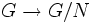# Conjugacy-distinguished element

Jump to: navigation, search

This article defines a property of elements in groups

## Definition

An element$g$ in a group$G$ is termed conjugacy-distinguished if whenever$h$ is an element of$G$ not conjugate to$g$, there exists a normal subgroup of finite index$N \le G$, such that the images of$g$ and$h$ under the quotient map$G \to G/N$ are not conjugate in$G/N$.

A group where every element is conjugacy-distinguished is termed a conjugacy-separable group.